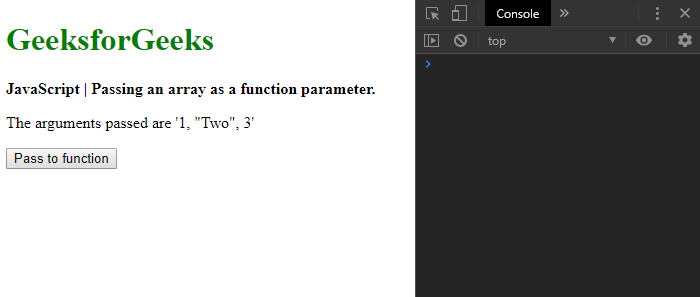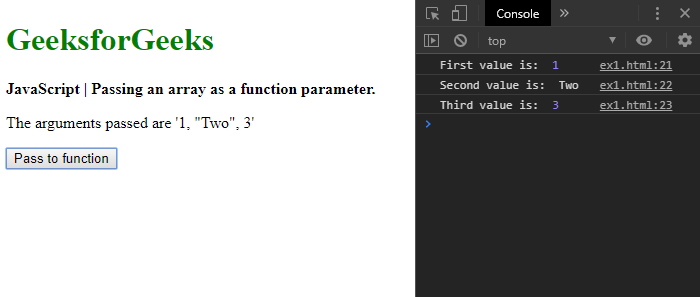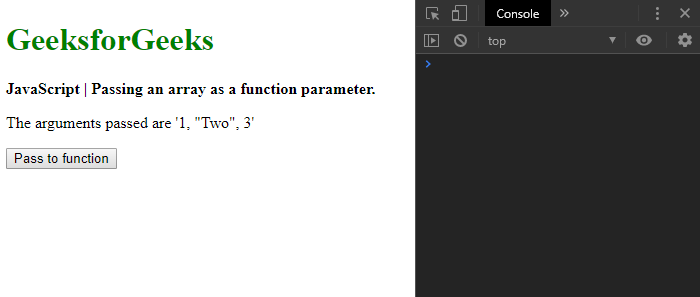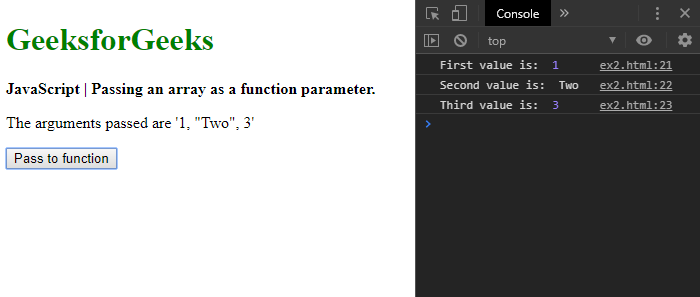Related Articles
How to pass an array as a function parameter in JavaScript ?
• Difficulty Level : Basic
• Last Updated : 26 Nov, 2019

Method 1: Using the apply() method: The apply() method is used to call a function with the given arguments as an array or array-like object. It contains two parameters. The this value provides a call to the function and the arguments array contains the array of arguments to be passed.

The apply() method is used on the function that has to be passed as the arguments array. The first parameter is specified as ‘null’ and the second parameter is specified with the arguments array. This will call the function with the specified arguments array.

Syntax:

 `arrayToPass = [1, ``"Two"``, 3]; ` ` `  `unmodifiableFunction.apply(``null``, arrayToPass); `

Example:

 ` ` `<``html``> ` ` `  `<``head``> ` `    ``<``title``> ` `        ``How to pass an array as a function ` `        ``parameter in JavaScript ? ` `    `` ` ` ` ` `  `<``body``> ` `    ``<``h1` `style``=``"color: green"``> ` `        ``GeeksforGeeks ` `    `` ` `     `  `    ``<``b``> ` `        ``JavaScript | Passing an array ` `        ``as a function parameter. ` `    `` ` `     `  `    ``<``p``> ` `        ``The arguments passed ` `        ``are '1, "Two", 3' ` `    `` ` `     `  `    ``<``button` `onclick``=``"passToFunction()"``> ` `        ``Pass to function ` `    `` ` `     `  `    ``<``script` `type``=``"text/javascript"``> ` `         `  `        ``function passToFunction() { ` `            ``arrayToPass = [1, "Two", 3]; ` `         `  `            ``unmodifiableFunction.apply(null, arrayToPass); ` `        ``} ` `     `  `        ``function unmodifiableFunction(a, b, c) { ` `            ``console.log("First value is: ", a); ` `            ``console.log("Second value is: ", b); ` `            ``console.log("Third value is: ", c); ` `        ``} ` `    `` ` ` ` ` `  ` `

Output:

• Before clicking the button:• After clicking the button:Method 2: Using the spread syntax: The spread syntax is used in place where zero or more arguments are expected. It can be used with iterators that expands in place where there may not be a fixed number of expected arguments (like function parameters).

The required function is called as given the arguments array using the spread syntax so that it would fill in the arguments of the function from the array.

Syntax:

 `arrayToPass = [1, ``"Two"``, 3]; ` ` `  `unmodifiableFunction(...arrayToPass); `

Example:

 ` ` `<``html``> ` ` `  `<``head``> ` `    ``<``title``> ` `        ``How to pass an array as a function ` `        ``parameter in JavaScript ? ` `    `` ` ` ` ` `  `<``body``> ` `    ``<``h1` `style``=``"color: green"``> ` `        ``GeeksforGeeks ` `    `` ` `     `  `    ``<``b``> ` `        ``JavaScript | Passing an array ` `        ``as a function parameter. ` `    `` ` `     `  `    ``<``p``> ` `        ``The arguments passed ` `        ``are '1, "Two", 3' ` `    `` ` `     `  `    ``<``button` `onclick``=``"passToFunction()"``> ` `        ``Pass to function ` `    `` ` `     `  `    ``<``script` `type``=``"text/javascript"``> ` `     `  `        ``function passToFunction() { ` `            ``arrayToPass = [1, "Two", 3]; ` `         `  `            ``unmodifiableFunction(...arrayToPass); ` `        ``} ` `     `  `        ``function unmodifiableFunction(a, b, c) { ` `            ``console.log("First value is: ", a); ` `            ``console.log("Second value is: ", b); ` `            ``console.log("Third value is: ", c); ` `        ``} ` `    `` ` ` ` ` `  ` `

Output:

• Before clicking the button:• After clicking the button:My Personal Notes arrow_drop_up
Recommended Articles
Page :